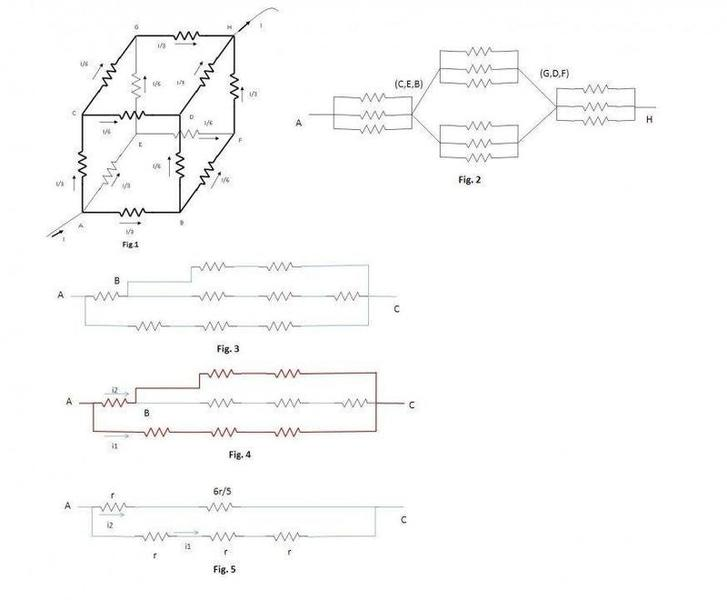# 12 Resistor cube problem

Q. It is a classical problem in current electricity .The resistor cube consists of 12 resistors, each having a value r. What is the total resistance between the two diagonally opposite corners labeled A and H on the cube?

Ans. The answer to this problem begins with that due to symmetry points E, B and C will be at same potential. Similarly again extending the argument of symmetry, the points G, D, and F will again be at same potential. Why these points will be at same potential is explained by the fact that due to symmetry each arm AC, AB, AE will have same current (I/3) which is equal to 1/3 of total current (I) entering the junction A. Similarly equal amount of current (I/3) will be leaving branches GH, FH, DH and combining at junction H. (See Fig.1)

Now using above symmetry concept circuit reduces to following (value of each resistor is r) (See Fig.2)This circuit can be easily solved.

I personally had a difficult time understanding how the symmetry leads to division of current I into three equal parts along AC, AB and AE, until I found this statement.

“The network is symmetric about the entry point A and exit point B. By symmetry, we mean that if the minimum number of identical resistances along the shortest paths between entry and exit points of the current is the same for two or more paths then those paths are symmetrical”Let’s call this law as “X”.

I also found a video solving above problem through this approach. The solution is as follows (Refer Fig.1) The possible shortest paths from A to H through C

A->C->G->H, A->C->D->H

Similarly, possible shortest paths from A to H through E

A->E->G->H, A->E->F->H

Finally, possible shortest paths from A to H through B

A->B->D->H, A->B->F->H

Here we see that shortest path from A to H through arms AC, AE, AB involves three resistors, hence the paths through these arms are symmetrical and equal current (I/3)will flow through arms AC, AE and AB.

Now coming to nodes B, C and D. Let us take the node B. From B the shortest path to H is B->F->H and B->D->H

Since the shortest path from B to H through arms BF and BD involves two resistors, hence the paths through these arms are symmetrical and equal current (I/6) will flow through BF and BD.

Applying same logic at node C and E we get current I/6 in branches CD and CG , EG and EF. Using Kirchhoff’s current law we can find the current in remaining branches. Now we may apply Kirchhoff’s voltage law by applying voltage source between the terminals A and H and considering any one path from A to H. We get the equivalent resistance as 5r/6.

When I am trying to apply the above mentioned law “X” in the following simple circuit (Refer Fig.3)to find the current in branches AB and AC ), I am getting wrong result.

Let’s see how. Since the shortest path from A to C through arms ABC and AC involve three resistors, hence the paths through these arms should be symmetrical which means i1=i2.(Refer Fig.4)

However, if we simplify the circuit bit we get the reduced circuit (Refer Fig.5).

Here clearly, i1 ≠i2.So where am I wrong .Have I misunderstood the law “X” or is it something else ?mfb
Mentor
When I am trying to apply the above mentioned law “X” in the following simple circuit (Refer Fig.3)to find the current in branches AB and AC ), I am getting wrong result.
I don't see how you apply "law X" to get figure 3. You seem to ignore some additional connections. You can use symmetry to identify different points with each other, but you cannot simply ignore connections (there is current in all resistors).

Sir
Could you please tell how to apply Law "X" to circuit given in figure 3.

mfb
Mentor
It is phrased in a confusing way, and I don't think it is useful here.

Anyway, the symmetry should be easy to see and verify. There is a three-fold symmetry corresponding to a rotation of the cube around AH, and a twofold symmetry by mirroring the whole cube (entry and exit are symmetric). The rotational symmetry is sufficient here.